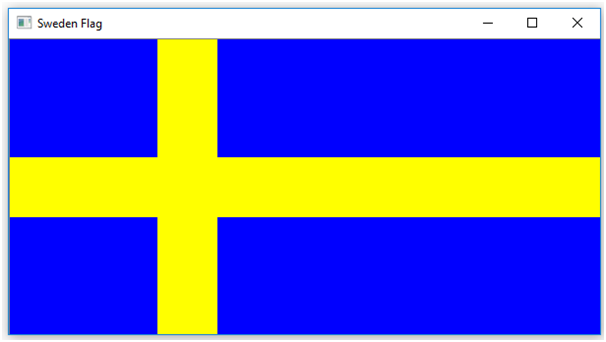# Drawing of flag Sweden | Image processing in Python

This is an example of image processing in python – here we are going to learn how to draw a flag of Sweden in python?
Submitted by Ankit Rai, on April 23, 2019

A colored image can be represented as a 3 order matrix. The first order is for the rows, the second order is for the columns and the third order is for specifying the color of the corresponding pixel. Here we use the BGR color format(because OpenCV python library works on BGR format, not on RGB), so the third order will take 3 values of Blue, Green, and Red respectively. The values of the rows and columns depending on the size of the image.

In Python programming language, we can use an OpenCV library named cv2. Python does not come with cv2, so we need to install it separately.

For Windows:

```    pip install opencv-python
```

For Linux:

```    sudo apt-get install python-opencv
```

Colour planes of BGR image:

Consider a BGR image array I then,

• I[:, :, 0] represents the Blue colour plane of the BGR image
• I[:, :, 1] represents the Green colour plane of the BGR image
• I[:, :, 2] represents the Red colour plane of the BGR image

The flag of Sweden consists of a yellow or gold Nordic Cross (i.e. an asymmetrical horizontal cross, with the crossbar closer to the hoist than the fly, with the cross extending to the edge of the flag) on a field of blue. The Nordic Cross design traditionally represents Christianity.

Steps:

First, we make a matrix of dimensions 300 X 600 X 3. Where the number of pixels of rows is 300, the number of pixels of columns is 600 and 3 represent the number of dimensions of the color coding in BGR format.

• Paint the complete image in blue. BGR code for blue is (255,0, 0,).
• Make the horizontal yellow bar. BGR code for yellow is (0,255, 255).
• Make the vertical yellow bar.

## Python code to draw flag of Sweden

```# import numpy library as np
import numpy as np

# import open-cv library
import cv2

# here image is of class 'uint8', the range of values
# that each colour component can have is [0 - 255]

# create a zero matrix of order 300x600 of 3-dimensions
image = np.zeros((300, 600, 3),np.uint8)

# fill whole pixels of dimensions
# with Blue colour.
image[:, :, 0] = 255;

# fill the pixels in range 120-180
# of row with Yellow colour
image[120:181, :, 1] = 255;
image[120:181, :, 2] = 255;
image[120:181, :, 0] = 0;

# fill the pixels in range 150 - 210
# of coloumn with Yellow colour
image[:, 150:211, 1] = 255;
image[:, 150:211, 2] = 255;
image[:, 150:211, 0] = 0;

# Show the image formed
cv2.imshow("Sweden Flag",image);
```

OutputPreparation